• Call Now

1800-102-2727•

# Screw Gauge - Definition, Working, Measurement & Errors

Is it possible to measure lengths smaller than millimeter by a scale whose smallest calibration is millimeter? You might think no, but we can indeed do this by making a few arrangements! Let’s see how in this article. For this the widely used instruments are vernier caliper and screw gauge. They also involve some degree of error. These errors come due to improper usage or wear and tear. While analysing the error and correcting it, we can have near perfect results.

## Screw GaugeTo measure the length even in the micrometer(μm=10-6 m) range, we can use a micrometer screw or screw gauge. This is used on the principle of a screw thread system. This is an accurately cut single threaded screw, rotated in a precisely fitted nut. Because of the screw thread arrangement, it has two types of motion: circular and linear. The arrangement moves along the axis of the screw. The linear distance moved by the screw in a complete rotation is called the pitch. It is also defined as the distance between two consecutive peaks or troughs of the thread. Usually, it is 1 mm. The circumference of the screw is divided into 100 or 50 equal parts. So, in a circular motion adding one point in thimble scale makes the screw advance or retract by 1100 mm or 150 mm. This is the least count in a micrometer screw or screw gauge which we can use to measure the smallest length accurately. That is why it is called the least count of a screw gauge.

Let’s say we have n divisions on the circumference. The pitch is p mm.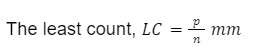## Measuring with a Screw Gauge

While measuring, the object to be measured is put between the anvil and the spindle. The length of the object is read through the main scale reading on the sleeve and screw reading on thimble scale reading matching with the datum line.

While measuring with a screw gauge we can stumble upon different errors.

## Zero Error in Screw Gauge

If the zero marks in the main scale and in the thimble scale don’t coincide at the time when the anvil and the spindle touch each other, it has zero error. Zero error is subtracted from the total reading we got previously.

In positive zero error(+Z) the thimble scale zero-mark advances beyond the main scale zero mark when the anvil and the spindle touch each other.

In negative zero error(-Z) the thimble scale zero mark is left behind the main scale zero mark when the anvil and the spindle touch each other.Zero error is also counted as any reading in the screw gauge

## Backlash Error in Screw Gauge

When the direction of rotation in the screw suddenly changes, the screw may continue to rotate but the screw itself will not move forward or backwards. So, the scale reading may change even by the actual movement of the screw itself. This is the backlash error. This error arises due to the wear and tear in screw threading after prolonged usage. To reduce this error, the screw must always be rotated, for a set of observations, in the same direction.

## Practice Problems of Screw Gauge

Question 1. In a screw gauge there are 100 divisions on the thimble scale and screw advances 1 mm in a complete rotation of the screw cap. In another screw gauge there are 200 divisions on the thimble scale and screw advances 1 mm in a complete rotation of the screw cap. Who is more precise in measurement?

Answer: The least count for the first screw gauge is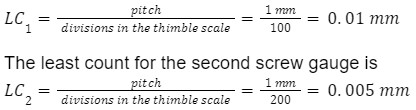Here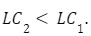So, the second screw gauge is more precise in measurement.

Question 2. In a screw gauge, when the anvil and spindle are touching each other the zero mark on the thimble scale matches with the zero mark of the main scale. There are 100 divisions on the thimble scale and screw advances 1 mm in a complete rotation of the screw cap. A rod of diameter D is measured with this screw gauge. It shows 3 in main scale reading and 15 on the thimble scale. What is the value of D ?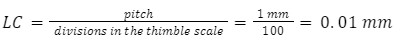The zero mark in thimble scale matches with the datum line when the spindle and the anvil touch each other. So, no zero error exists. Z=LC×0=0 mm

∴D=MSR + xLC -Z=3+15×0.01-0=3.15 mm

Question 3. In a screw gauge, when the anvil and spindle are touching each other the zero mark on the thimble scale goes slightly beyond the zero mark of the main scale. At that moment the 55th division mark in thimble scale matches perfectly with the datum line. There are 100 divisions on the thimble scale and screw advances 1 mm in a complete rotation of the screw cap. A rod of diameter D is measured with this screw gauge. It shows 5 in main scale reading and 12 on the thimble scale. What is the value of D ?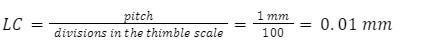The zero mark in thimble scale goes past the datum line when the spindle and the anvil touch each other. So, a positive zero error exists. Z=LC×55=0.01 mm×55=0.55 mm

∴D=MSR + xLC - Z=5+12×0.01-0.55=4.57 mm

Question 4. In a screw gauge, when the anvil and spindle are touching each other the zero mark on the thimble scale goes left behind the zero mark of the main scale. At that moment the 15th division mark in thimble scale matches perfectly with the datum line. There are 50 divisions on the thimble scale and screw advances 1 mm in a complete rotation of the screw cap. A rod of diameter D is measured with this screw gauge. It shows 3 in main scale reading and 22 on the thimble scale. What is the value of D ?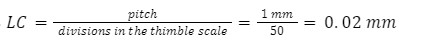The zero mark in the thimble scale is left behind the datum line when the spindle and the anvil touch each other. So, a negative zero error exists. Z=LC×15=-0.02 mm×15=-0.3 mm

∴D=MSR + xLC -Z=3+22×0.02+0.3=3.74 mm

## FAQs of Screw Gauge

Question 1. What do you understand by the pitch of a screw gauge?

Answer: The pitch of a screw gauge is the distance travelled by the screw for a complete rotation of the screw head.

Question 2. What do you understand by the least count of a screw gauge?

Answer: The least count is the pitch divided by the number of divisions on the thimble scale. It is the distance travelled by the screw head for completing the rotation amounting to one division on the thimble scale. The least count is the minimum distance or length measurable by the screw gauge.

Question 3. In a screw gauge the screw advances 1 mm in one complete rotation of the thimble scale cap. There are 100 divisions in the thimble scale. The least count is

a. 0.01 mm
b. 0.02 mm
c. 0.1 mm
d. 0.2 mm

Question 4. A 4.92 mm diameter wire when measured by a faulty screw gauge gives a reading of 5.01 mm. What will be the reading while measuring a 5.01 mm diameter rod?

a. 4.92 mm
b. 5.01 mm
c. 5.10 mm
d. none of the above

Answer: 5.10 mm = 5.01+0.09 (due to zero error)

## NCERT Class 11 Physics Chapters

 Physical World Units and Measurements Motion in a Straight Line Motion in a Plane Laws of Motion Work Energy and Power Particles and Rotational Motion Gravitation Mechanical Properties of Solids Mechanical Properties in Liquids Thermal Properties of Matter Thermodynamics Kinetic Theory Oscillations WavesTalk toour expert
Resend OTP Timer =
By submitting up, I agree to receive all the Whatsapp communication on my registered number and Aakash terms and conditions and privacy policy